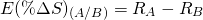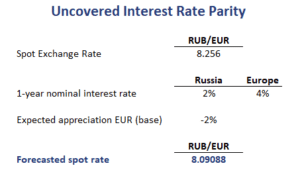# Uncovered Interest Rate Parity

Uncovered interest rate parity is used when capital flows are restricted or when there are no currency forward contracts that can be used. In that case, arbitrage is not taking place. Because there is no arbitrage, the covered interest parity may not hold. In that case, we make use of the uncovered interest rate parity.

In what follows, we discuss the uncovered interest rate parity formula, implement an uncovered interest rate parity example and discuss what happens if the forward rate is an unbiased predictor of future spot rates. An Excel example can be downloaded at the bottom of the page.

## Uncovered interest rate parity formula

Let’s have a look at the uncovered interest rate parity formula. Let’s assume we have a quote A/B, then the the uncovered interest rate parity formula iswhere RA is the interest rate in the country of the price currency and RB is the interest rate in the country of the base currency. Thus, the expected change in the spot rate equals the interest rate differential between both countries.

## Uncovered interest rate parity example

Let’s consider an example. The following table uses the uncovered interest rate parity to forecast the future exchange rate using both countries’ interest rates.Clearly, applying the uncovered interest rate parity formula is very trivial. It only requires subtracting the interest rate from the price currency from the interest rate of the base currency.

## Forward rate parity

If the forward rate is equal to the future expected spot rate, then we say that the forward rate is an unbiased predictor of the future spot rate. If this is the case, then we refer to this condition as forward rate parity

## Summary

The uncovered interest rate parity refers to a situation where arbitrage does not insure that the forward premium or discount on a currency forward contract offsets the differences between interest rates between countries.